Courses

# Context Free Language Practice Quiz - 1

## 10 Questions MCQ Test RRB JE for Computer Science Engineering | Context Free Language Practice Quiz - 1

Description
This mock test of Context Free Language Practice Quiz - 1 for Computer Science Engineering (CSE) helps you for every Computer Science Engineering (CSE) entrance exam. This contains 10 Multiple Choice Questions for Computer Science Engineering (CSE) Context Free Language Practice Quiz - 1 (mcq) to study with solutions a complete question bank. The solved questions answers in this Context Free Language Practice Quiz - 1 quiz give you a good mix of easy questions and tough questions. Computer Science Engineering (CSE) students definitely take this Context Free Language Practice Quiz - 1 exercise for a better result in the exam. You can find other Context Free Language Practice Quiz - 1 extra questions, long questions & short questions for Computer Science Engineering (CSE) on EduRev as well by searching above.
QUESTION: 1

### Which of the following languages is generated by the given grammar?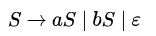Solution: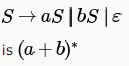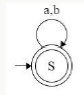QUESTION: 2

### Consider the following context-free grammars;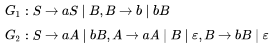Solution:

G1 results in strings b, ab, bb, aab, abb, bbb, ... i.e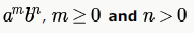(and because only a's are not possible but only b's are)

G2 result in strings a, b, aa, ab, bb, aaa, aab, abb, bbb ... i.e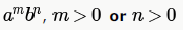(or because only b's is possible
b,bb,bbb, , only a's are possible)

QUESTION: 3

### Consider the following languages: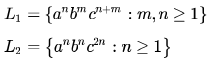Which one of the following is TRUE?

Solution: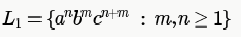is Context-free language

(push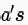into stack, then push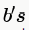into stack , read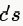and pop, when noleft on stack, keep readingand pop, when no c's left in input , and stack is empty, then accepted).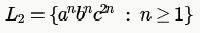is Context-sensitive language and not context-free. (cannot implemented by one stack)

QUESTION: 4

A context-free grammar is ambiguous if

Solution:

an ambiguous grammar produces more than one parse tree for any string.. that's B..

*Multiple options can be correct
QUESTION: 5

02. Choose the correct alternatives (more than one may be correct) and write the corresponding letters only: (xix) Context-free languages are

Solution:

Context Free languages are not closed under intersection and complementation.

QUESTION: 6

Choose the correct alternatives (more than one may be correct) and write the corresponding letters only:

If G is a context free grammar and  is a string of lengthl  in L(G), how long is a derivation of ω in G, if G is in Chomsky normal form?

Solution:

Chomsky Normal Form (If all of its production rules are of the form):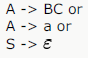where A, B and C are nonterminal symbols, a is a terminal symbol (a symbol that represents a constant value), S is the start symbol, and ε is the empty string. Also, neither B nor C may be the start symbol, and the third production rule can only appear if ε is in L(G), namely, the language produced by the context-free grammar G.

Applying productions of the first form will increase the number of nonterminals from k to k+1 since you replace one
nonterminal (-1) with two nonterminals (+2) for a net gain of +1 nonterminal. Since you start with one nonterminal, this means you need to do l-1 productions of the first form. You then need l more of the second form to convert the
nonterminals to terminals, giving a total of l+(l-1) = (2l-1) productions.

QUESTION: 7

Which of the following definitions below generate the same language as L , where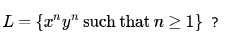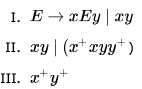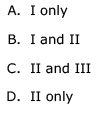Solution:

In the other two you can have any number of x and y. There is no such restriction over the number of both being equal.

QUESTION: 8

If L1 and L2 are context free languages and  a regular set, one of the languages below is not necessarily a context free language. Which one?

Solution:

CFL's are not closed under intersection.

QUESTION: 9

Define a context free languages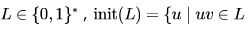for some υ in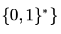( in other words, (L) is the set of prefixes of L).

Let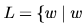is nonempty snd has an equal number of 0's and 1's}

Then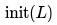is

Solution:

Because for any binary string of 0's and 1's we can append another string to make it contain equal
number of 0's and 1's. i.e., any string over {0,1} is a prefix of a string in L.

Example:

01111 - is prefix of 011110000 which is in L.
1111- is prefix of 11110000 which is in L.
01010- is prefix of 010101 which is in L.

QUESTION: 10

Context-free languages are closed under:

Solution:

Cfl are not closed under intersection and complement now choose the correct option so b)union and klenne closure